The KDE Procedure

Convolutions

The formulas for the binned estimator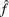in the previous subsection are in the form of a convolution product between two matrices, one of which contains the bin counts, the other of which contains the rescaled kernels evaluated at multiples of grid increments. This section defines these two matrices explicitly, and shows thatis their convolution.

Beginning with the weighted univariate case, define the following matrices: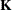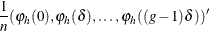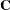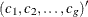The first thing to note is that many terms inare negligible. The term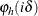is taken to be 0 when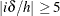, so you can define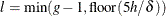as the maximum integer multiple of the grid increment to get nonzero evaluations of the rescaled kernel. Here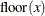denotes the largest integer less than or equal to x.

Next, let p be the smallest power of 2 that is greater than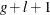,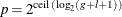where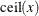denotes the smallest integer greater than or equal to x.

Modifyas follows: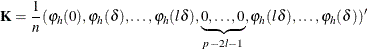Essentially, the negligible terms ofare omitted, and the rest are symmetrized (except for one term). The whole matrix is then padded to size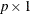with zeros in the middle. The dimension p is a highly composite number—that is, one that decomposes into many factors—leading to the most efficient fast Fourier transform operation (see Wand; 1994).

The third operation is to pad the bin count matrix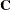with zeros to the same size as: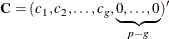The convolution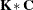is then amatrix, and the preceding formulas show that its first g entries are exactly the estimates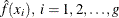.

For bivariate smoothing, the matrixis defined similarly as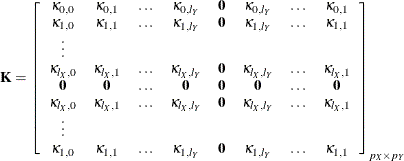where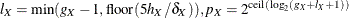, and so forth, and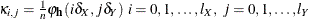.

The bin count matrixis defined as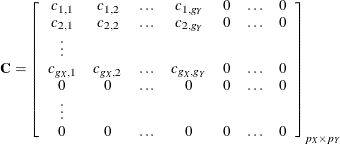As with the univariate case, the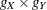upper-left corner of the convolutionis the matrix of the estimates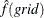.

Most of the results in this subsection are found in Wand (1994).